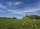# Pythagorean theorem - examples - page 27

1. KLM triangleFind the length of the sides of the triangle KLM if m = 5cm height to m = 4.5 cm and size MKL angle is 70 degrees.
2. BricklayerHow much do we pay for a bricklayer laying a pavement in a square room with a diagonal of 8 m, if 1 sqm with work will cost for CZK 420?
3. Circle annulusThere are 2 concentric circles in the figure. Chord of larger circle 10 cm long is tangent to the smaller circle. What are does annulus have?
4. ParatrooperAfter the parachute is opened, the paratrooper drops to the ground at a constant speed of 2 m/s, with the sidewinding at a steady speed of 1.5 m/s. Find: a) the magnitude of its resulting velocity with respect to the ground, b) the distance of his land fr
5. Tangent spheresA sphere with a radius of 1 m is placed in the corner of the room. What is the largest sphere size that fits into the corner behind it? Additional info: Two spheres are placed in a corner of a room. The spheres are each tangent to the walls and floor and
6. Center of line segmentCalculate the distance of the point X [1,3] from the center of the line segment x = 2-6t, y = 1-4t ; t is .
7. The gardenThe garden has the shape of a rectangular trapezium. The bases have lengths of 27 meters and 36 meters, the trapezoid's height is 12 meters. Calculate how much fence will cost this garden if one meter costs 1.5 €?
8. Distance problem 2A=(x,2x) B=(2x,1) Distance AB=√2, find value of x
9. Distance problemA=(x, x) B=(1,4) Distance AB=√5, find x;
10. Right isosceles triangleRight isosceles triangle has an altitude x drawn from the right angle to the hypotenuse dividing it into 2 equal segments. The length of one segment is 5 cm. What is the area of the triangle?
11. Rectangular fieldA rectangular field has a diagonal of length 169m. If the length and width are in the ratio 12:5. Find the dimensions of the field, the perimeter of the field and the area of the field.
12. Pile of sandA large pile of sand has been dumped into a conical pile in a warehouse. The slant height of the pile is 20 feet. The diameter of the base of the sand pile is 31 feet. Find the volume of the pile of sand.
13. Right 24Right isosceles triangle has an altitude x drawn from the right angle to the hypotenuse dividing it into 2 unequal segments. The length of one segment is 5 cm. What is the area of the triangle? Thank you.
14. CincinnatiA map is placed on a coordinate grid. Cincinnati located at (5,4) and San Diego is located at (-10, -3). How far apart is Cincinnati from San Diego on the map? Round to the nearest tenth.

We apologize, but in this category are not a lot of examples.
Do you have an interesting mathematical example that you can't solve it? Enter it, and we can try to solve it.

To this e-mail address, we will reply solution; solved examples are also published here. Please enter e-mail correctly and check whether you don't have a full mailbox.

Pythagorean theorem is the base for the right triangle calculator.